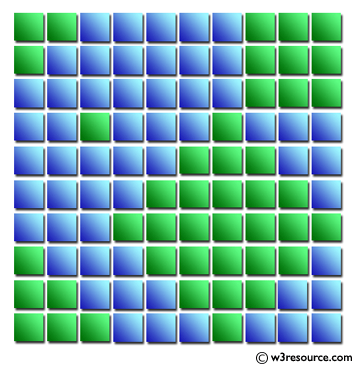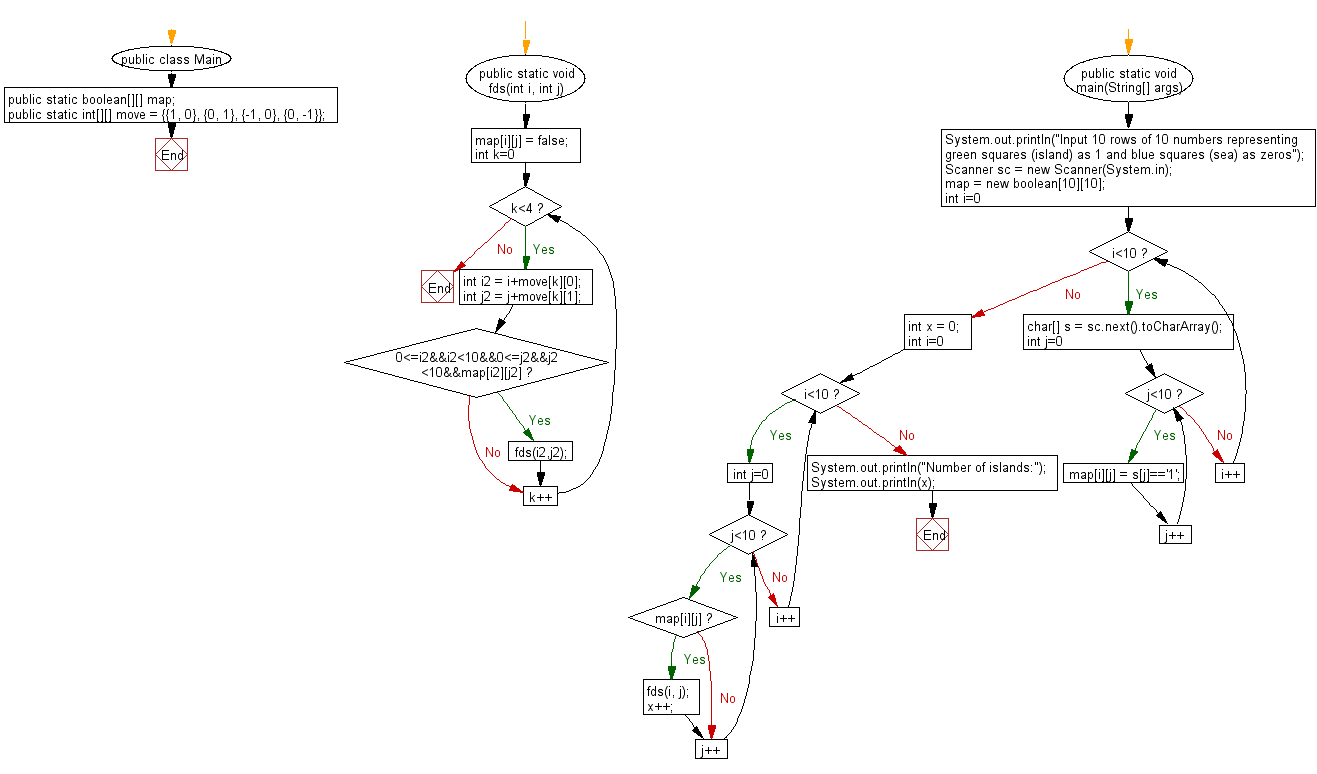# Java Exercises: Read the mass data and find the number of islands

## Java Basic: Exercise-237 with Solution

There are 10 vertical and horizontal squares on a plane. Each square is painted blue and green. Blue represents the sea, and green represents the land. When two green squares are in contact with the top and bottom, or right and left, they are said to be ground. The area created by only one green square is called "island". For example, there are five islands in the figure below.
Write a Java program to read the mass data and find the number of islands.

Input:
A single data set is represented by 10 rows of 10 numbers representing green squares as 1 and blue squares as zeros.
Output: For each data set, output the number of islands.

Pictorial Presentation:Sample Solution:

Java Code:

``````  import java.util.Scanner;

public class test {

public static boolean[][] map;

public static int[][] move = {{1, 0}, {0, 1}, {-1, 0}, {0, -1}};

public static void fds(int i, int j){
map[i][j] = false;
for(int k=0;k<4;k++){
int i2 = i+move[k];
int j2 = j+move[k];
if(0<=i2&&i2<10&&0<=j2&&j2<10&&map[i2][j2])fds(i2,j2);
}
}
public static void main(String[] args) {
System.out.println("Input 10 rows of 10 numbers representing green squares (island) as 1 and blue squares (sea) as zeros");
Scanner sc = new Scanner(System.in);
map = new boolean;
for(int i=0;i<10;i++){
char[] s = sc.next().toCharArray();
for(int j=0;j<10;j++){
map[i][j] = s[j]=='1';
}
}
int x = 0;
for(int i=0;i<10;i++){
for(int j=0;j<10;j++){
if(map[i][j]){
fds(i, j);
x++;
}
}
}
System.out.println("Number of islands:");
System.out.println(x);
}
}
```
```

Sample Output:

```Input 10 rows of 10 numbers representing green squares (island) as 1 and blue squares (sea) as zeros
1100000111
1000000111
0000000111
0010001000
0000011100
0000111110
0001111111
1000111110
1100011100
1110001000
Number of islands:
5
```

Flowchart:Java Code Editor:

What is the difficulty level of this exercise?

﻿

## Java: Tips of the Day

Directory Content:

Java allows you to get the names of all subdirectories and files in a folder as an array, which can then be sequentially viewed.

```import java.io.*;

public class ListContents {
public static void main(String[] args) {
File file = new File("//home//user//Documents/");
String[] files = file.list();

System.out.println("Listing contents of " + file.getPath());
for(int i=0 ; i < files.length ; i++)
{
System.out.println(files[i]);
}
}
}
```